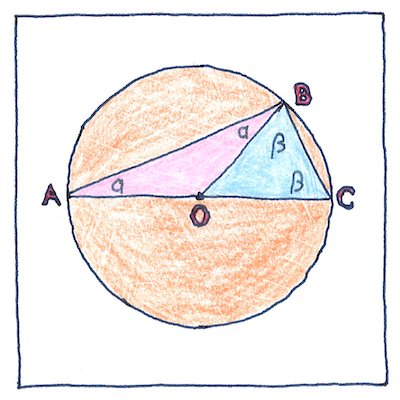# 546 BCE

## The book of science

Tom Sharp

 Thales of Miletus geometry

## Thales’ theorem

• Take the ends of the diameter of a circle, A and C,
• and consider any other point on the circle, B.
• then the triangle ABC is a right triangle.
• Consider the two isosceles triangles OAB and OBC.
• The base angles of an isosceles triangle are equal,
• and the three angles of a triangle add up to 180 degrees.
• Therefore, two α plus two β equals 180 degrees,
• so it is clear that α plus β equals 90 degrees,
• which is a right angle.

## Dante’s theorem

• Solomon chose wisdom
• so he could be an adequate king,
• not knowledge of the number
• of moving spirits in heaven,
• nor whether a prime mover exists,
• nor whether a necessary conclusion
• can be drawn from a necessary premise
• and a contingent premise,
• nor whether a triangle
• can be constructed in a semicircle
• that does not have a right angle.

## Thales’ ideal

• A point has no diameter,
• a line no width.
• A straight line has no deviation,
• a circle only one curvature.
• We do not assume these ideals;
• we do not pretend them.
• We say that any point, line, or circle
• drawn on wax or clay
• is only an approximation,
• only a crude representation.
• When our loved ones are with us,
• they can never be perfect.
• But true love is true,
• and can never deviate.

Like the Pythagorean theorem, Thales’ theorem was known by Indian and Babylonian mathematicians before Thales learned of it.

Dante’s theorem is a paraphrase of Paradiso Canto 13, lines 94-102.

See also in The book of science:

Readings on wikipedia: